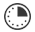# Graph Smile

JRDAssist
Middle School and High School Teacher

Pairs of students will survey other students in the school and then graph the results, as well as come to conclusions based on the information.

##Length of Time: 3 Class Periods

### Common Core Alignment

CCSS.Math.Content.6.SP.A.1 - Recognize a statistical question as one that anticipates variability in the data related to the question and accounts for it in the answers. For example, "How old am I?" is not a statistical question, but "How old are the students in my school?" is a statistical question because one anticipates variability in students' ages.

CCSS.Math.Content.6.SP.A.2 - Understand that a set of data collected to answer a statistical question has a distribution which can be described by its center, spread, and overall shape.

CCSS.Math.Content.6.SP.B.4 - Display numerical data in plots on a number line, including dot plots, histograms, and box plots.

CCSS.Math.Content.6.SP.B.5 - Summarize numerical data sets in relation to their context, such as by:

## Objectives & Outcomes

The students will be able to create statistical questions, survey others, display and analyze the results, and report conclusions in a presentation to the class.

## Materials Needed

Prepare ahead of time:    Find a graph showing results of a survey for the opening to the lesson.

## Procedure

### Opening to Lesson

• Display a graph showing the results of a survey.  It could be a simple one-question line or bar graph.  Ask students if they know the different parts of the graph: title, intervals, etc.
• Ask:  What information does the graph show?  What are the results of the survey?  What can you interpret from the graph?  What conclusions could you make?   Who would benefit from knowing the results?

Allow for responses and discussion.

### Body of Lesson

DAY ONE

#### Modeling

• Tell students they will be creating similar surveys and reporting the results and conclusions.
• They will answer the same types of questions based on results of their created surveys.
• Review the different types of graphs with students:  bar, line, etc.  (You may use a worksheet for student practice before beginning the activity.)

#### Guided Practice

• Pair students.  Tell each pair they must create 3 to 5 related questions to ask other students in the school.  Give an example of two questions that may be related:  What time do you go to bed at night?  What time do you wake up?
• Once the questions are created, the students will use the survey with 25 students.  They will record the results.
• The student pairs must create a data-collection page to record the results as well.  The surveys will be conducted and results used the next day.
• The teacher should review the survey questions before students are permitted to approach other students.  In addition, if possible try to prevent similar or repeated patterns of questions.

DAY TWO

• Pairs of students meet to organize and discuss the results of the surveys.
• They must decide on which type of graph or graphs to use to display the results using the poster board.
• They begin compiling the data, find the mean, range, and other information which will be helpful for interpreting the information.
• The two students will then use the information to write out a short report which will be orally presented to the class.
• Next, create a display board to creatively present the findings, using images, a title, etc.
• Allow the students at least one full class period to complete the project once they gathered all of their data.
• Schedule students’ presentations the next day.

DAY THREE

• Each pair of students receives between 3 to 5 minutes to report their findings and interpretations to the class, drawing conclusions, etc.
• The presentations should answer the questions, and more, posed in the opening to the lesson.
• Remind students NOT to simply report the results.
• Assess the students based on a rubric prepared ahead of time.

#### Independent Practice

• Teacher-created or commercial handouts for practice with graphing, including interpreting graphs, the mean, range, etc.

### Closing

• Ask:  What difficulties were there in surveying other students?  What were the variables?  What would they have done differently? (Or other questions based on the experience.)

## Assessment & Evaluation

Create a rubric to be used for the class activity and presentation.  Use teacher-created or other materials to quiz or test students on creating and interpreting graphs using a given set of data.

## Modification & Differentiation

Students create survey questions to be used outside of class with adults, family members, or others.  Students work alone on the data collection.  No oral reports, all information is presented in a written report.  Use of Excel spreadsheet program for students to create the graphs.

## Related Lesson Plans

##### Prices and Percentages

Using a current list of prices for food and clothing, the students will practice math skills related to percentages.

##### Angles in Nature

Students will take a walk outside with their protractor and measure the angles in nature. They will record the angles that they find in branches, trees, bushes, flowers, etc… and then determine the supplementary angle.

##### Interest-ing Math

Students will learn about simple interest and how to calculate the real cost of a loan, credit card, and other types of borrowing.

##### Unknown Volumes

Finding the volumes of miscellaneous-sized containers using a known total measurement of one container.  They will do this in as few steps as possible.